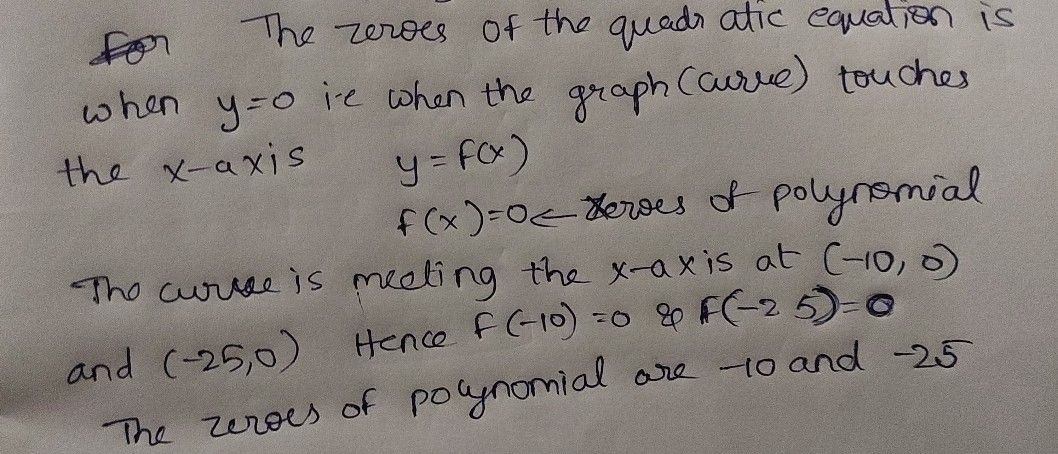Symbol
ProblemIdentify the zeros of each quadratic function. $1\right)$ $4y$ $2\right)$ $20$ $5$ $10$ $x$ $35-30-2$ $-20-15$ $0$ $25$ $10$ $15$ $+0$ $15$ $-20$ $25$ $zeros$ : $2$
10th-13th grade
Algebra
SolutionQanda teacher - abdulhaqStudent
??Qanda teacher - abdulhaq
We are not allowed to solve multiple questions in one session. Please ask this question in a separate session.Student
wtv